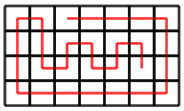CSES - Grid Path Construction
• Time limit: 1.00 s
• Memory limit: 512 MB

Given an n \times m grid and two squares a=(y_1,x_1) and b=(y_2,x_2), create a path from a to b that visits each square exactly once.

For example, here is a path from a=(1,3) to b=(3,6) in a 4 \times 7 grid:# Input

The first input line has an integer t: the number of tests.

After this, there are t lines that describe the tests. Each line has six integers n, m, y_1, x_1, y_2 and x_2.

In all tests 1 \le y_1,y_2 \le n and 1 \le x_1,x_2 \le m. In addition, y_1 \neq y_2 or x_1 \neq x_2.

# Output

Print YES, if it is possible to construct a path, and NO otherwise.

If there is a path, also print its description which consists of characters U (up), D (down), L (left) and R (right). If there are several paths, you can print any of them.

# Constraints

• 1 \le t \le 100
• 1 \le n \le 50
• 1 \le m \le 50

# Example

Input:

5
1 3 1 1 1 3
1 3 1 2 1 3
2 2 1 1 2 2
2 2 1 1 2 1
4 7 1 3 3 6


Output:

YES
RR
NO
NO
YES
RDL
YES
RRRRDDDLLLLLLUUURDDRURDRURD